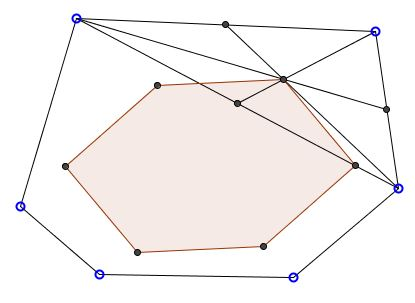# Construction of ParahexagonWhat is it? A Mathematical Droodle

Explanation### Construction of ParahexagonParahexagon is a hexagon whose opposite sides are parallel and equal. This is an analogue of Parallelogram, but with six sides. The term I believe has been coined by Kasner and Newman in their Mathematics and the Imagination. (Eves designates the polygons parpolygons.) The applet attempts to demonstrate the following construction of a Parahexagon.

Start with any hexagon. Each triple of consecutive vertices forms a triangle. The centers of the successive triangles necessarily form a parahexagon.

Indeed, let the vertices of the given hexagon be denoted as $P_{1},$ $P_{2},$ $P_{3},$ $P_{4},$ $P_{5},$ and $P_{6}.$ Consider the centers of two consecutive triangles described above, say, $P_{1}P_{2}P_{3}$ and $P_{2}P_{3}P_{4}.\,$ The triangles share a side, viz., $P_{2}P_{3}.\,$ Denote its midpoint as $M.$ In $\Delta P_{1}P_{2}P_{3},$ the center lies on the median $P_{1}M$ and divides it in the ratio $2:1$ counting from $P_{1}.$ Similarly, in $\Delta P_{2}P_{3}P_{4},$ the center lies on the median $P_{4}M$ and divides it in the ratio $2:1$ counting from $P_{4}.$ It follows, that in $\Delta P_{1}MP_{4},$ the line joining the two centers is parallel to $P_{1}P_{4}$ and is equal $1/3$ of the latter.

The opposite side of the hexagon under investigation joins the centers of triangles $P_{4}P_{5}P_{6}$ and $P_{5}P_{6}P_{1}.$ It, too, is parallel to $P_{1}P_{4}$ and equals its third.

That result admits a generalization that also covers the Varignon parallelogram.

Polygons with opposite sides parallel but not necessarily equal still have interesting properties.

### References

1. H. Eves, A Survey of Geometry, Allyn and Bacon, 1972
2. E. Kasner and J. Newman in their Mathematics and the Imagination, Dover \$Publications (March 28, 2001)